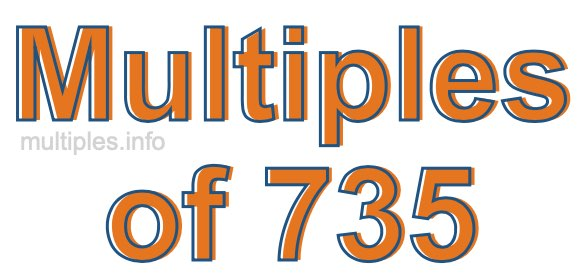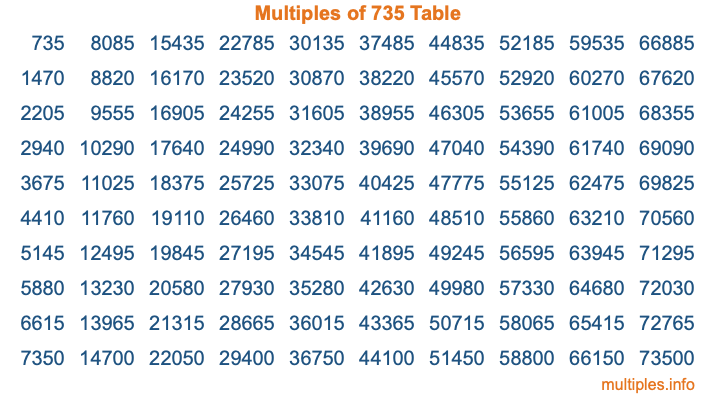Multiples of 735Welcome to the Multiples of 735 page. Here we will first teach you everything you will ever need to know about the multiples of 735, and then give you a study guide summary of everything we taught you to make sure you remember it all. Use this page to look up facts and learn information about the multiples of 735. This page will make you a multiples of seven hundred thirty-five expert!

Definition of Multiples of 735
Multiples of 735 are all the numbers that when divided by 735 equal an integer. Each of the multiples of 735 are called a multiple. A multiple of 735 is created by multiplying 735 by an integer.

Therefore, to create a list of multiples of 735, you start with 1 multiplied by 735, then 2 multiplied by 735, then 3 multiplied by 735, and so on for as long as you want. Thus, the list of the first five multiples of 735 is 735, 1470, 2205, 2940, and 3675. To see a larger list of multiples of 735, see the printable image of Multiples of 735 further down on this page. We also have a category where you can choose any nth multiple of 735.

Multiples of 735 Checker
The Multiples of 735 Checker below checks to see if any number of your choice is a multiple of 735. In other words, it checks to see if there is any number (integer) that when multiplied by 735 will equal your number. To do that, we divide your number by 735. If the the quotient is an integer, then your number is a multiple of 735.

Is  a multiple of 735?

Least Common Multiple of 735 and ...
A Least Common Multiple (LCM) is the lowest multiple that two or more numbers have in common. This is also called the smallest common multiple or lowest common multiple and is useful to know when you are adding our subtracting fractions. Enter one or more numbers below (735 is already entered) to find the LCM.

Check out our LCM Calculator if you need more details about the Least Common Multiple or if you need the LCM for different numbers for adding and subtraction fractions.

nth Multiple of 735
As we stated above, 735 is the first multiple of 735, 1470 is the second multiple of 735, 2205 is the third multiple of 735, and so on. Enter a number below to find the nth multiple of 735.

th multiple of 735

Multiples of 735 vs Factors of 735
735 is a multiple of 735 and a factor of 735, but that is where the similarities end. All postive multiples of 735 are 735 or greater than 735. All positive factors of 735 are 735 or less than 735.

Below is the beginning list of multiples of 735 and the factors of 735 so you can compare:

Multiples of 735: 735, 1470, 2205, 2940, 3675, etc.

Factors of 735: 1, 3, 5, 7, 15, 21, 35, 49, 105, 147, 245, 735

As you can see, the multiples of 735 are all the numbers that you can divide by 735 to get a whole number. The factors of 735, on the other hand, are all the whole numbers that you can multiply by another whole number to get 735.

It's also interesting to note that if a number (x) is a factor of 735, then 735 will also be a multiple of that number (x).

Multiples of 735 vs Divisors of 735
The divisors of 735 are all the integers that 735 can be divided by evenly. Below is a list of the divisors of 735.

Divisors of 735: 1, 3, 5, 7, 15, 21, 35, 49, 105, 147, 245, 735

The interesting thing to note here is that if you take any multiple of 735 and divide it by a divisor of 735, you will see that the quotient is an integer.

Multiples of 735 Table
Below is an image of the first 100 multiples of 735 in a table. The table is in chronological order, column by column. The first column has the first ten multiples of 735, the second column has the next ten multiples of 735, and so on.The Multiples of 735 Table is also referred to as the 735 Times Table or Times Table of 735. You are welcome to print out our table for your studies.

Negative Multiples of 735
Although not often discussed or needed in math, it is worth mentioning that you can make a list of negative multiples of 735 by multiplying 735 by -1, then by -2, then by -3, and so on, to get the following list of negative multiples of 735:

-735, -1470, -2205, -2940, -3675, etc.

Multiples of 735 Summary
Below is a summary of important Multiples of 735 facts that we have discussed on this page. To retain the knowledge on this page, we recommend that you read through the summary and explain to yourself or a study partner why they hold true.

There are an infinite number of multiples of 735.

A multiple of 735 divided by 735 will equal a whole number.

735 divided by a factor of 735 equals a divisor of 735.

The nth multiple of 735 is n times 735.

The largest factor of 735 is equal to the first positive multiple of 735.

735 is a multiple of every factor of 735.

735 is a multiple of 735.

A multiple of 735 divided by a divisor of 735 equals an integer.

735 divided by a divisor of 735 equals a factor of 735.

Any integer times 735 will equal a multiple of 735.

Multiples of a Number
Here you can get the multiples of another number, all with the same attention to detail as we did for multiples of 735 on this page.

Multiples of
Multiples of 736
Did you find our page about multiples of seven hundred thirty-five educational? Do you want more knowledge? Check out the multiples of the next number on our list!